## OCP Benchmarks

Optimal Control Problem (OCP) solvers are a powerful tool for designing control strategies that optimize a given objective function subject to constraints. They are widely used in engineering, economics, finance, and management, and are applied to a wide range of problems to find optimal solutions that minimize costs, maximize profits, reduce risk, or achieve other desired outcomes. The control strategy is typically represented as a function of time that maps the control input (manipulated variable) to the state of the system. The state of the system is determined by a set of differential equations, and the objective function is a measure of the system performance over a time horizon.

#### Exercise

Objective: Set up and solve several OCP benchmarks. Create a program to optimize and display the results. Estimated Time (each): 30 minutes

#### Aircraft Control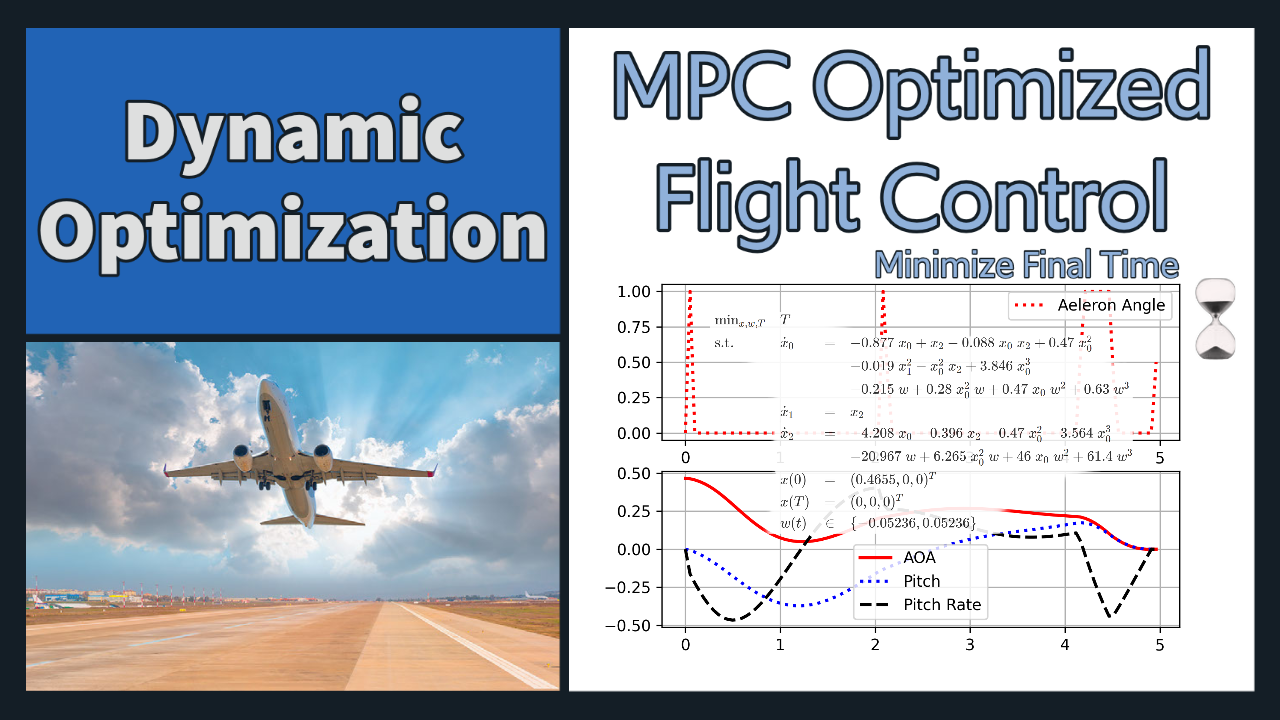#### Batch Reactor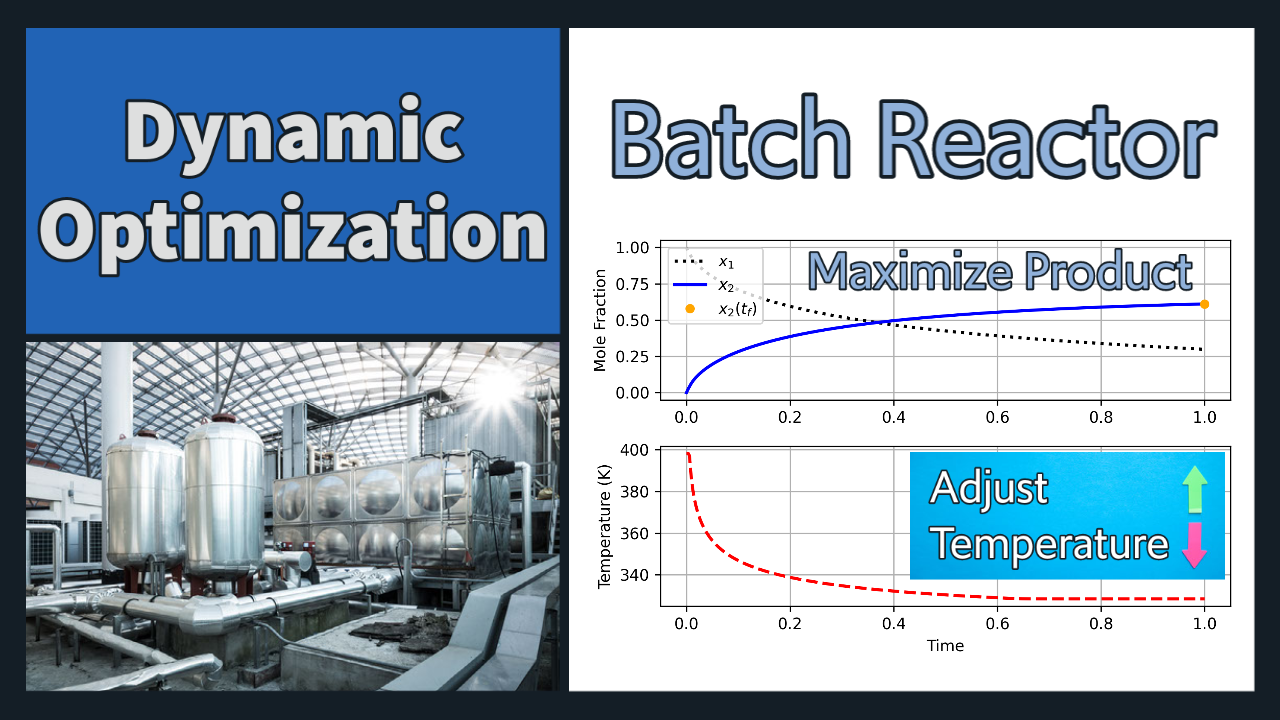#### Bryson-Denham Problem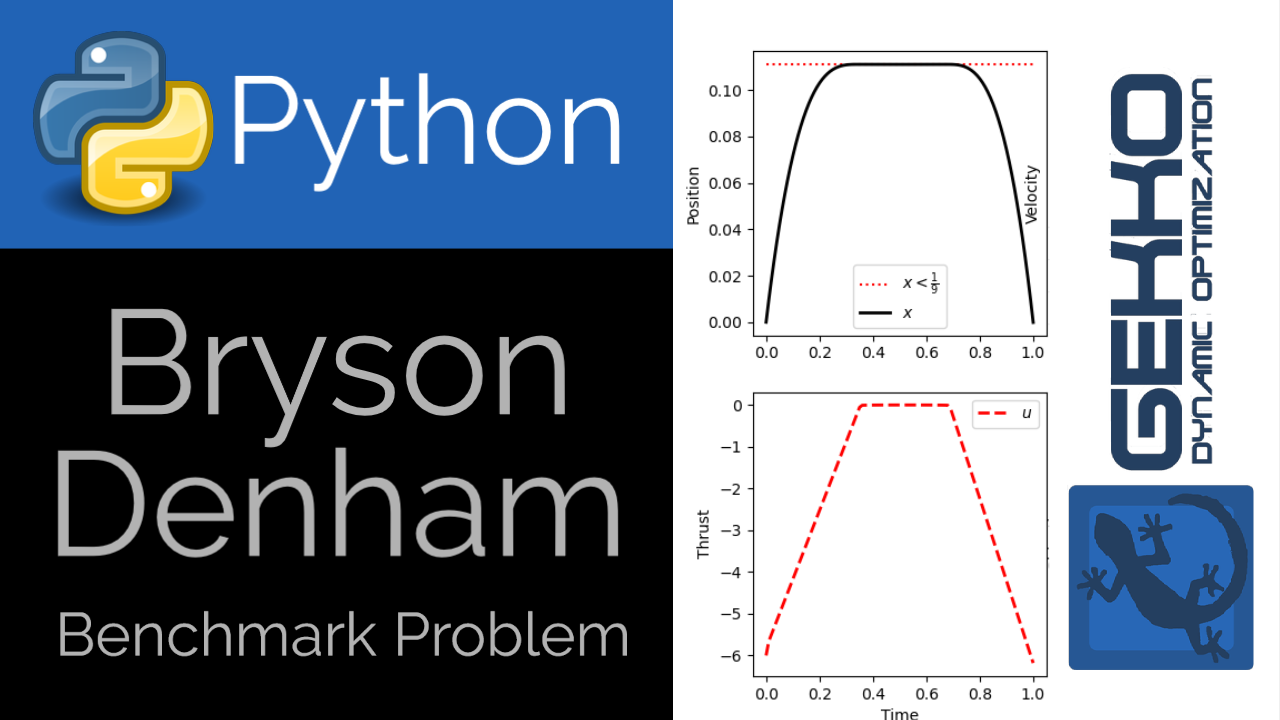#### Drone Flight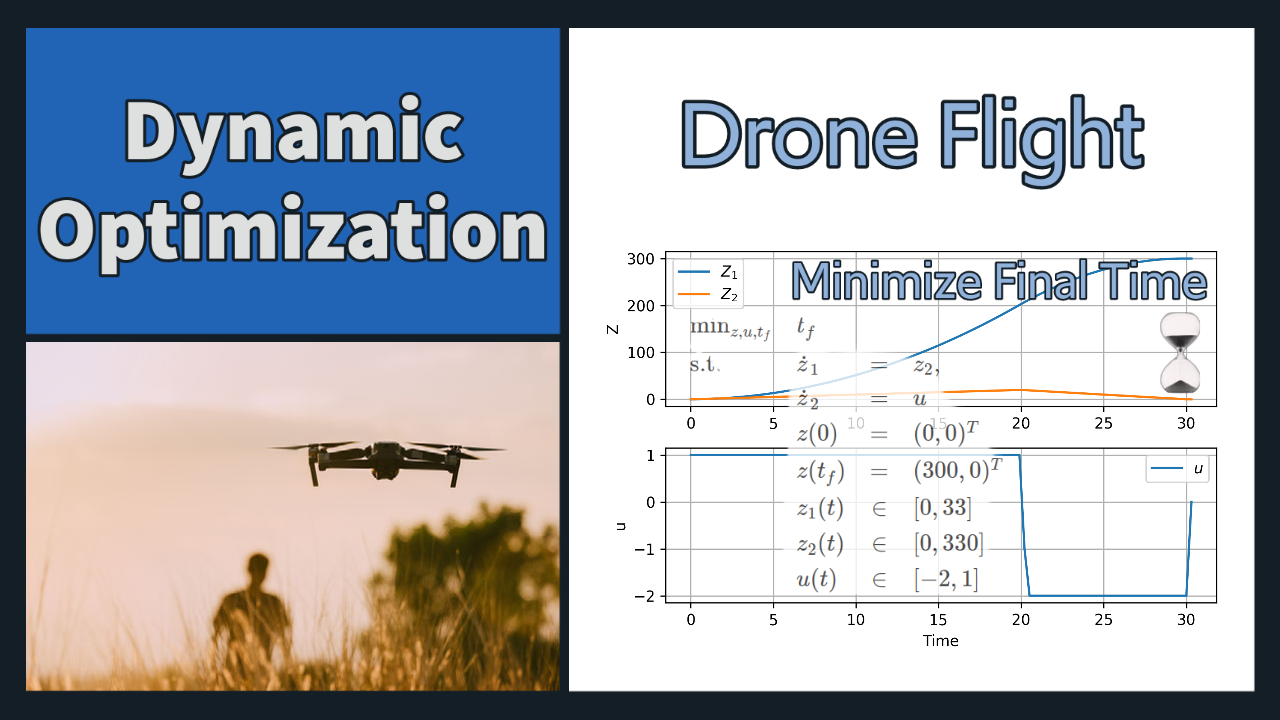#### Hanging Chain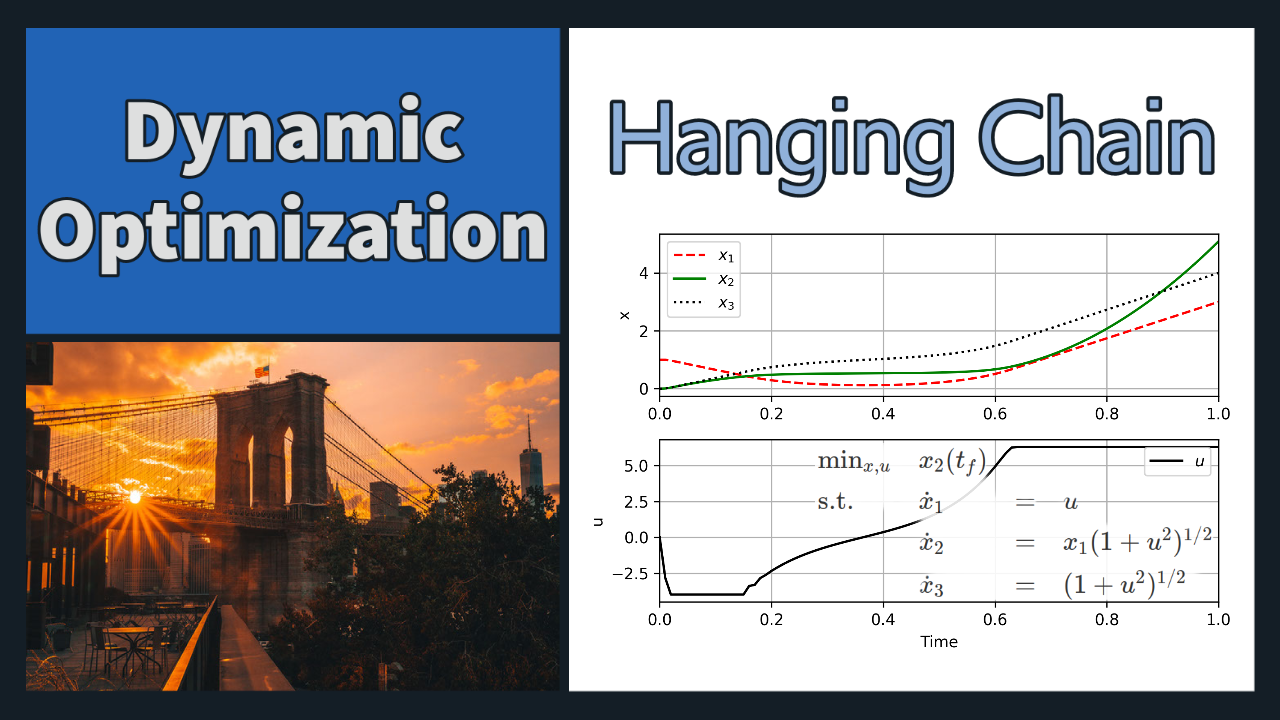#### Integral Objective (Luus)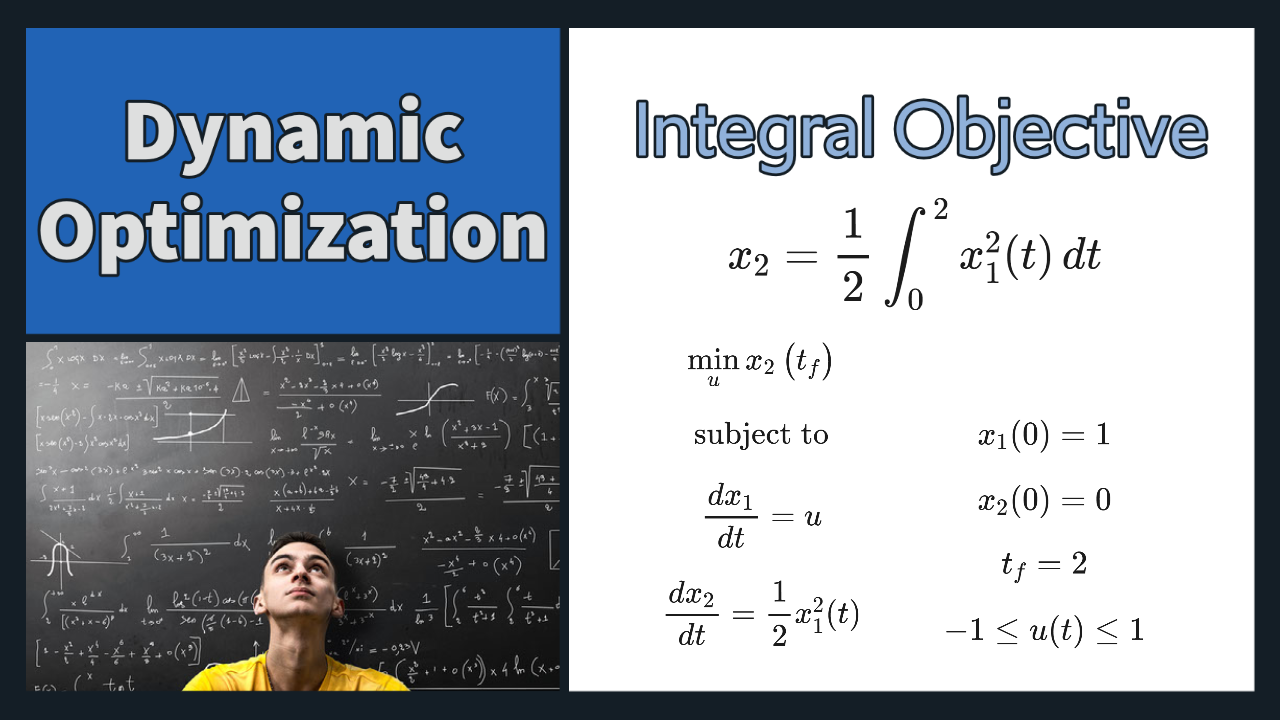#### Maximize Profit (Commercial Fishery)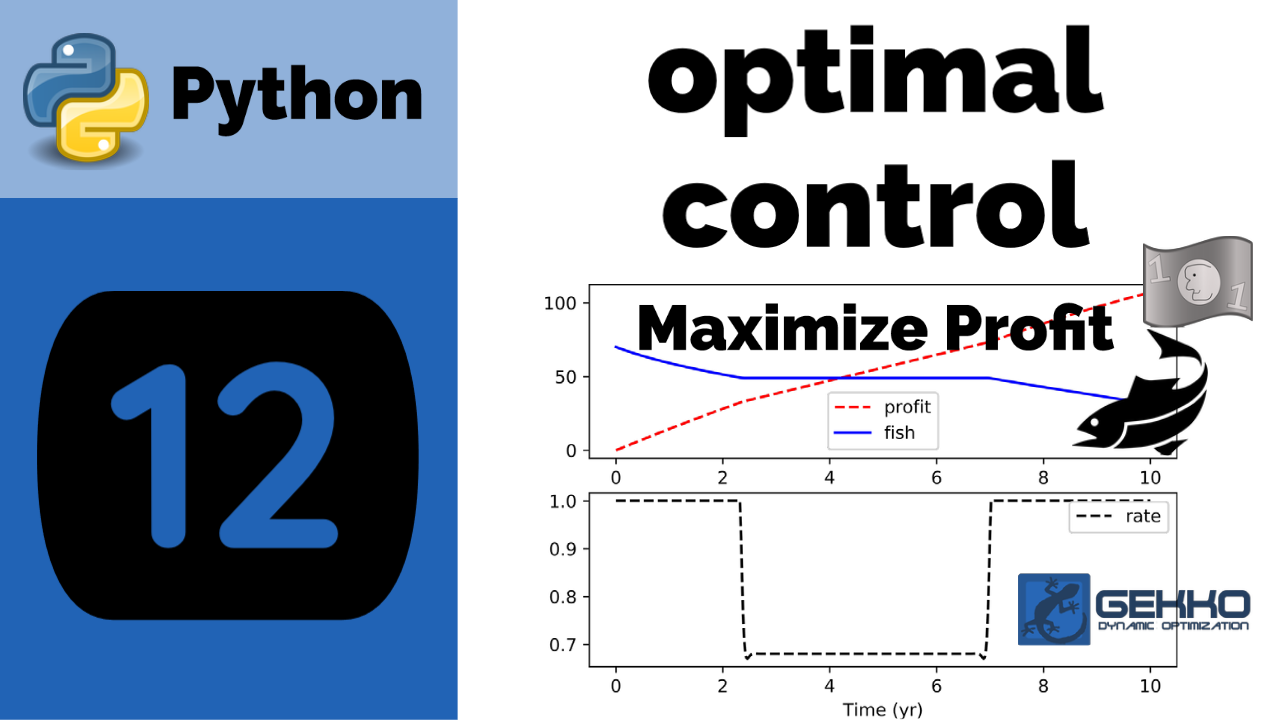#### Minimize Final Time (Jennings)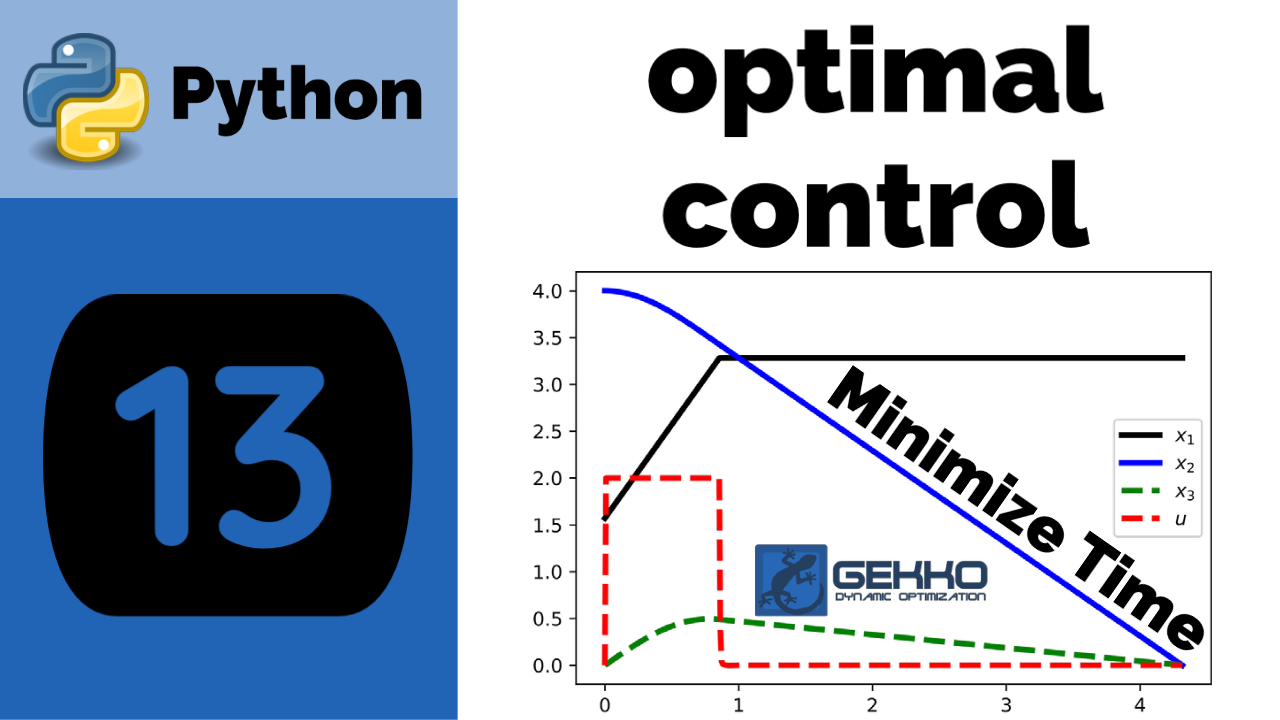#### Oil Shale Pyrolysis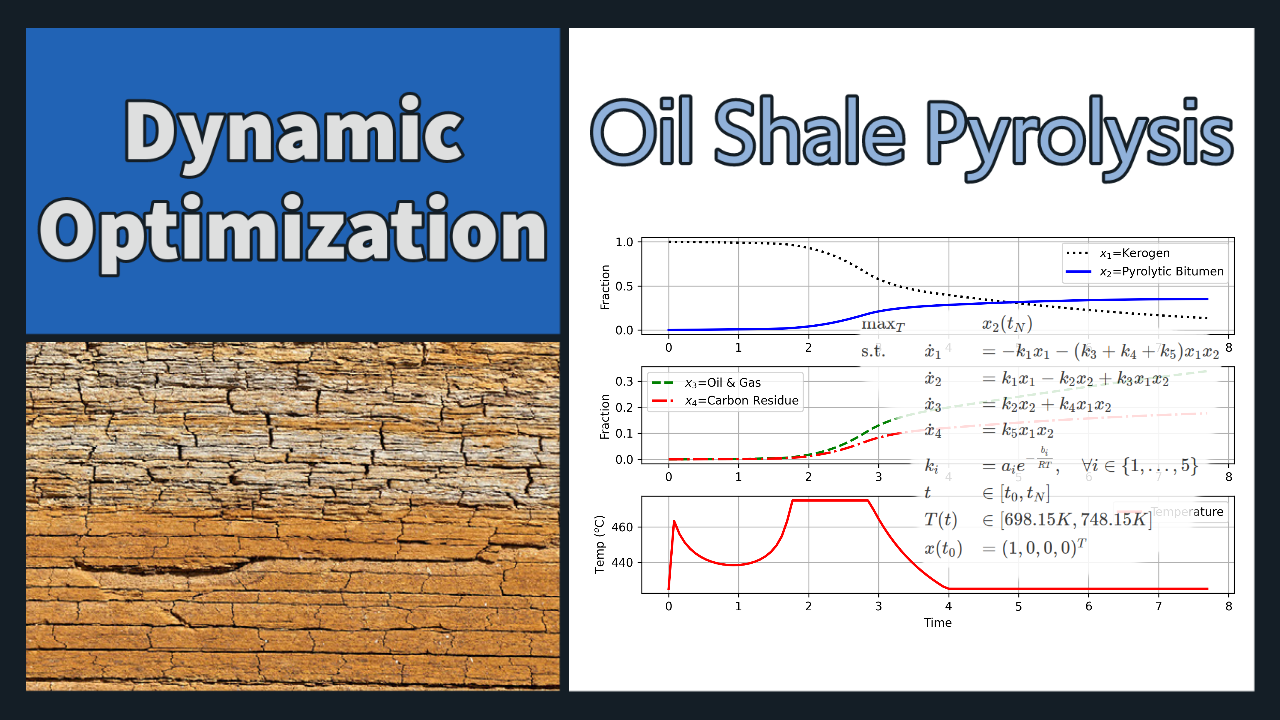#### Aly-Chan Singular Control Problem

• Solve the following nonlinear and constrained problem.
• The objective is to minimize final state x3(pi/2) by adjusting the value of u.
• Compare to the exact solution of u(t)= -sin(t).Dynamic Optimization Aly-Chan Solution in MATLAB and Video

import numpy as np
import matplotlib.pyplot as plt
from gekko import GEKKO

m = GEKKO()

nt = 1001
t = np.linspace(0,np.pi/2,nt)
m.time = t

# Variables
x1 = m.Var(value=0)
x2 = m.Var(value=1)
x3 = m.Var(value=0)

u = m.MV(value=0,ub=1,lb=-1)
u.STATUS = 1
u.DCOST = 0

p = np.zeros(nt)
p[-1] = 1.0
final = m.Param(value=p)

# Equations
m.Equation(x1.dt()==x2)
m.Equation(x2.dt()==u)
m.Equation(2*x3.dt()==x2**2-x1**2)

# Objective Function
m.Obj(x3*final)

m.options.IMODE = 6
m.options.NODES = 4
m.solve()

plt.figure(1)
plt.subplot(2,1,1)
plt.plot(m.time,x1.value,'k:',lw=2,label=r'$x_1$')
plt.plot(m.time,x2.value,'b-',lw=2,label=r'$x_2$')
plt.plot(m.time,x2.value,'k-',lw=2,label=r'$x_3$')
plt.subplot(2,1,2)
plt.plot(m.time,u.value,'r--',lw=2,label=r'$u$')
plt.plot(t,-np.sin(t),'k:',lw=2,label='Exact')
plt.legend(loc='best')
plt.xlabel('Time')
plt.ylabel('Value')
plt.show()

#### Aly Singular Control ProblemAly Singular Control Solution in MATLAB and Video

See estimation example using the same model to explain estimator objectives.

#### Catalyzed ReactionCatalyzed Reaction Optimized in MATLAB and Video

#### References

1. Beal, L.D.R., Hill, D., Martin, R.A., and Hedengren, J. D., GEKKO Optimization Suite, Processes, Volume 6, Number 8, 2018, doi: 10.3390/pr6080106. Article
2. Hedengren, J. D. and Asgharzadeh Shishavan, R., Powell, K.M., and Edgar, T.F., Nonlinear Modeling, Estimation and Predictive Control in APMonitor, Computers and Chemical Engineering, Volume 70, pg. 133–148, 2014. Article
3. Aly G.M. and Chan W.C. Application of a modified quasilinearization technique to totally singular optimal problems. International Journal of Control, 17(4): 809-815, 1973.